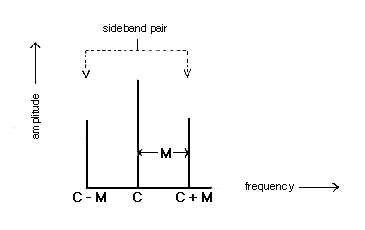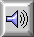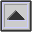AMPLITUDE MODULATION (AM)

The process of varying the AMPLITUDE of a sound, often periodically. An example of AM is the violinist's TREMOLO, where the amplitude of the vibrating string is rapidly altered by a movement of the bow.

In radio transmission, the process involves the MODULATION of a CARRIER wave's amplitude by the program SIGNAL being transmitted. See: MODULATED CARRIER.

By analogy to the radio sense of the term, amplitude modulation is used in electronic and computer SOUND SYNTHESIS to refer to a similar process where both the carrier and the modulating signals are in the audio range, or the latter in the SUBAUDIO range. If both are pure SINE TONEs, then the SPECTRUM produced consists of the carrier frequency plus a single pair of SIDEBANDs, equally spaced about the carrier at a frequency distance equal to the modulating frequency. If the modulating signal is 8 Hz or lower, the result is a TREMOLO, whereas above about 20 Hz the TIMBRE of the tone is changed. In between these two frequencies, a transition from one effect to the other occurs. If the carrier consists of other PARTIALs, then a pair of sidebands is produced about each of them. Generally, the process is used for timbral synthesis.

Ring modulation is a special case of amplitude modulation where the two signals (carrier and modulator) are multiplied together and produce only the sum and difference sidebands. See MODULATION for a sound example.Amplitude modulation spectrum of two sine waves, carrier C and modulator M, showing the addition of a pair of sidebands, C + M and C - M, around the carrier.

The amplitude of the modulating signal controls the amplitude of the carrier frequency, as well as that of the sidebands, in the resulting spectrum. The strength of the modulation is called the depth of modulation or percentage of modulation and is defined as 100 x (A - B)/(A + B) where A and B are the maximum and minimum peak-to-peak amplitudes of the modulated signal respectively. The depth of modulation in the signal shown is 100%.An amplitude modulated signal (below) where the frequency ratio of the carrier and modulating signals (above) is 10:1.Sound Example: AM signal with 500 Hz carrier, 50 Hz modulator, raising the depth of modulation from 0 to 100%.

home